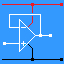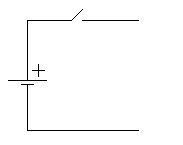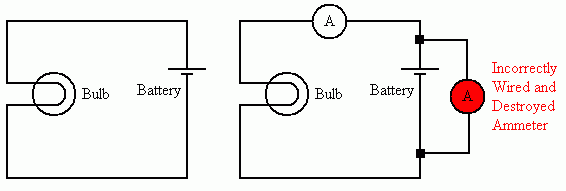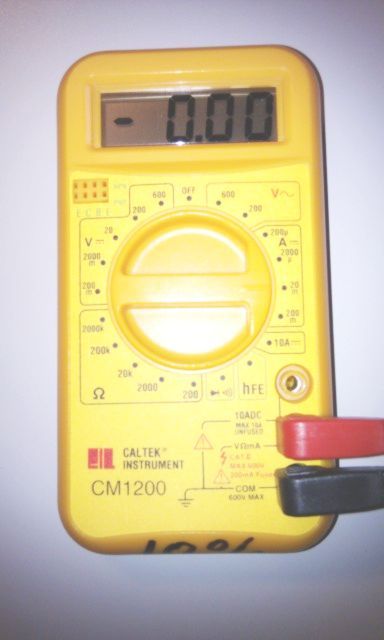RANDOM PAGE

SITE SEARCH

LOG
IN

HELP

# Measuring Current

This is the AQA version closing after June 2019. Visit the the version for Eduqas instead.

a

## Current - Measured in Amps

In a conductor, a current is a net flow of electrons. For a current to flow, there must be ...

1. A complete circuit. In this circuit, current can NOT flow.2. A potential difference attracting or repelling the electrons.
b

## Currents at JunctionsKirchoff's Current Law.

At a junction, the number of electrons ( amps ) entering the junction is equal to the number of electrons leaving the junction. This is just like cars at road junctions.

In this diagram, 160mA flows through the battery and A1. At the junction, 100mA goes up and you can work out that 60mA turns right.

Kirchoff's Voltage Law.

The sum of the voltages round a closed loop is equal to sum of the battery or power supply voltages.

In this example, across R1 there is 3 V and across R2 there is 6 V.

3 V + 6 V = 9 V

The battery voltage is 9 V so the rule applies to this circuit.

c

## Ammeter Facts

• Ammeters measure the current THROUGH a component. Connect the ammeter in series with the device being measured.
• Voltmeters measure the potential difference (voltage) ACROSS a component. Connect the voltmeter in parallel with the device being measured.

## An Ideal Ammeter

• has zero volts across it
• has a resistance of zero Ohms
• does not affect the circuit it is measuring.
• real ammeters have resistances close to zero.
• Therefore very large currents can flow through the ammeter if it is wired up wrongly.
• Most ammeters are protected by a fuse.

## Measuring Current

### CORRECT

How the experts do it!

### WRONG

How the twonks do it!

Twonk is also the noise made by the pointer in an analogue ammeter smashing itself against the end stop shortly before the meter burns out.

The ammeter must be placed in SERIES with the component who's current needs to be measured.

Break the circuit by removing a wire and replace the wire with the ammeter. In this way, the current flows through the ammeter and can be measured.## Making Safe use of MultimetersMany beginners play multimeter roulette. If they are lucky, they only blow the fuse inside the meter. It is also possible to destroy the meter. To play this game, connect the meter before setting it to the correct range. with the circuit switched on, randomly turn the range knob until the meter is destroyed or the fuse blows Clueless! You are most likely to destroy the meter when trying to measure current. Remember that the current you are measuring must flow through the meter. Set the meter to the correct range before connecting it up. Set the meter to the correct range before switching on the circuit. If measuring voltage, set the meter to the highest voltage you are likely to encounter. Later you can switch to lower voltage settings if necessary. If you are measuring current, set the meter to the highest current you are likely to encounter. Later you can switch to lower current settings if necessary. Resistance can only be measured if the resistor is not wired up in a circuit.

reviseOmatic V3     Contacts, ©, Cookies, Data Protection and Disclaimers Hosted at linode.com, London